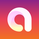Education

# RISK TO REWARD 📚 An Educational Write-up on How to Find This

BITSTAMP:BTCUSD   Bitcoin / U.S. Dollar
12903 views
Introduction:
This illustration explains the minimum Risk-To-Reward ratio needed based on your average win-rate while using a fixed % risk amount.

"Risk-To-Reward ratio": The ratio of what you stand to lose compared to win.

"Fixed % Risk": A static % amount of your total account balance at risk per trade.

"Fixed Dollar Risk": A static \$ amount at risk per trade. Regardless of account size fluctuations.

"Win-rate": The % out of all trades that are winners.

Steps:
1. Before being able to determine what Risk-To-Reward is acceptable to use, you will need to create a baseline measurement of your strategy's performance.

2. To create this baseline, you will need to backtest your strategy and obtain its current average win-rate.

3. This can be done using your pre-determined entry logic with a fixed stop-loss/take-profit offset amount.
(Adjusting your entry logic prior to finishing a round of backtesting may produce skewed results. Do not "cherry-pick" trades as that will lead to false results.)

4. Based on the resulting average win-rate you can then find the minimum Risk-To-Reward ratio you should be using.

5. Backtest again using the more optimal Risk-To-Reward ratio and repeat this step until the most optimal backtest results are obtained.

Here is the formula for determining your Average win-rate after you have tallied the wins/losses of your backtest:
#W = Number of winning trades
#L = Number of losing trades

(#W / (#W + #L)) * 100 = your average win rate %

-----

Introduction to Fixed Dollar Risk:
We have found it common for people to use the logic of fixed dollar risk amounts when calculating win-rates needed to break even, but then to use a fixed % risk in practice.

This simple-to-make mistake can lead to account erosion over time due to the way compounding works.

The fixed dollar approach uses relatively simple math for breaking even as shown below.

Example:
3 losing trades followed by 1 winning trade using 1:3 risk-to-reward achieves breakeven (ignoring trading fees and slippage)

This risk-to-reward ratio itself implies the win-rate needed (lose \$100 three times, win \$300 once, you break even).

The fixed dollar amount risk doesn't deal with compounding. As such, its logic cannot be used for fixed %.

-----

Using Fixed Percentage Risk:
Fixed % uses a more complicated and less apparent method for calculating how to break even. As shown in our illustration, if you take three losses in a row you won’t break even after your next win.

Fixed % is always dealing with the same % of your current balance. So as your balance decreases, the total dollar amount risked is less, and the total dollar amount gained with each win is reduced.

Thus, strings of losses require additional wins compared to the fixed dollar approach.

The fixed % method ensures against account erosion by showing the minimum win-rate needed to use each risk-to-reward ratio.

MATH NOTE: We used a simplified method for finding the minimum win-rate to make this useful and generally applicable. Our method is based on a given risk-to-reward ratio and assumes the max number of losses in a row to produce a minimum win-rate, it does not factor in all different possible loss strings and their probability.

-----

WHY USE FIXED % !?:
The question one will have at this point is, "Why to use fixed % if it is so F'ing complicated!?"

The answer to that is simple. Despite being more complicated, fixed % is actually objectively better by almost every other measure.

With fixed % you generally perform better than fixed dollar during strings of losses and wins. As with fixed %, you lose less as you go down (because you only ever lose 1% of your balance), and you gain more as you go up (because of your winnings compounding).

Not only that, but you also perform better even when losses and wins are more scattered, as you can see on the chart below.

-----

Conclusion:

Fixed % is more complicated than fixed dollar... to say the least.
However, it is none-the-less superior in most instances.

Use the logic above while using fixed % risk, since if you use fixed dollar logic but use fixed % in practice you will underperform your theoretical results.

If there are any major flaws in our logic/approach please let us know in the comments as of course, we are looking to provide as accurate instructional writeups as possible!
Comment: ~

After all of the positive feedback from the community on this post, we tried following it up with something just as interesting and this is what we came to the conclusion of:
• If your win-rate isn't allowing you to use an R:R you feel comfortable with, one solution is to search for a positively correlated market that produces better results while using the same strategy.

Here is our post on how to look for a better ROI:

All the support has been appreciated everyone! Feel free to reach out with requests or suggestions.
🧠
👀
👃
👅

🔥Our Indicators🚒
http://www.alphabotsystem.com/indicator-suite

⚰️Product Support👨‍⚕️
http://www.discord.gg/y3MeHmNWhere this is a good guide, it is not correct. The artical states a risk to reward to ratio of 1:X, then goes on to use percentage.
If you are risking a 1% of your account ballance (maximum anyone should be risking) with an account balance of \$100,000, first trade risk is \$1000, for a lose, the second risk would be \$990.
if L1+L2+L3+L4+L5 = \$4900 with the 6th trade risking \$950. so R2R is \$950:4900 1:5.15.
If risk is a value not a percentage i.e. \$1000 per trade, it does not matter if you win the first or last trade. the reward will be the same.
Watch this video very useful for understanding R2R https://www.youtube.com/watch?v=9uvTqMDh_CU